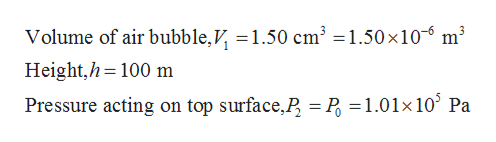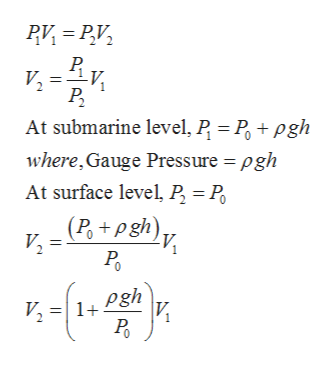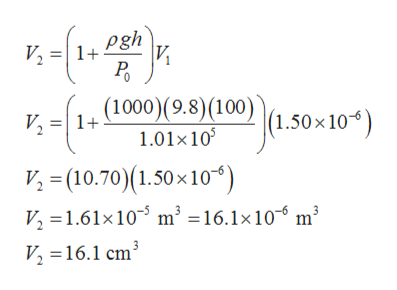# An air bubble has a volume of 1.50 cm3 when it is released by a submarine 1.00 x 102 m below the surface of a lake. What is the volume of the bubble when it reaches the surface? Assume the temperature and the number of air molecules in the bubble remain constant during its ascent.

Question
8 views

An air bubble has a volume of 1.50 cm3 when it is released by a submarine 1.00 x 102 m below the surface of a lake. What is the volume of the bubble when it reaches the surface? Assume the temperature and the number of air molecules in the bubble remain constant during its ascent.

check_circle

Step 1

Given,help_outlineImage TranscriptioncloseVolume of air bubble,V, =1.50 cm² =1.50×10-6 m³ Height,h=100 m Pressure acting on top surface,P, = P, =1.01x10° Pa %3D fullscreen
Step 2

By using Boyle’s equation between submarine and water surface and denoting submarine level as 1 and surface level as 2 then calculation can be done as follows,help_outlineImage TranscriptioncloseRV, = P,V, V, =v, P, At submarine level, P = P, + pgh where, Gauge Pressure = pgh At surface level, P = P, (P, +pgh). V, = P. pgh V, =| 1+ P fullscreen
Step 3

Since submarine is placed at 100 m below the above surface due to its situation the...help_outlineImage Transcriptionclosepgh = 1+ |И V2 P. (1000)(9.8)(100) 1.01×10 V, =| 1+ |(1.50×10*) V; = (10.70)(1.50×10*) V, =1.61×10 m³ =16.1×10- m³ V, =16.1 cm³ fullscreen

### Want to see the full answer?

See Solution

#### Want to see this answer and more?

Solutions are written by subject experts who are available 24/7. Questions are typically answered within 1 hour.*

See Solution
*Response times may vary by subject and question.
Tagged in

### Other